Version:

This documentation is not for the latest stable Salvus version.This tutorial is presented as Python code running inside a Jupyter Notebook, the recommended way to use Salvus. To run it yourself you can copy/type each individual cell or directly download the full notebook, including all required files.# Lamb's Problem

Copy
%matplotlib inline

# This line helps with tab-completion of the simple_config objects.
# The IPython/Jupyter project default to a differnet inference based
# tab completion engine which unfortunately does not yet fully work
# with SalvusFlow. This is completely optional and a convenience
# option.
%config Completer.use_jedi = False

## Introduction

An accurate solution to the wave equation is a requirement for a wide variety of seismological research. In this tutorial, we will validate the accuracy of SalvusCompute by comparing numerically calculated seismograms to semi-analytical solutions of Lamb's Problem in 2-D. In addition to giving us confidence in the synthetic data we will use in future tutorials, it also gives us a chance to gently learn some of the key features of the Salvus API.

Lamb's problem is concerned with the behavior of the elastic wave equation in the presence of a half-space bounded by a free-surface condition. In our solution we expect both direct arrivals and those reflected from the free-surface, along with a contribution from the 2-D Rayleigh wave. To validate the solutions generated with Salvus, we will compare our results with semi-analytical ones computed using EX2DDIR. We'll consider a half-space bounded at $y=2000$, and excite waves using a Ricker source with a center frequency of 15 Hz. This setup keeps compute times very low, while also allowing for a fair amount of wavelengths to propagate within our domain. To get started, let's first import all the Python tools we'll need.

# Standard Python packages
import os
import toml
import numpy as np
import matplotlib.pyplot as plt

# Workflow management.
from salvus.flow import api

# Specific objects to aid in setting up simulations.
from pyasdf import ASDFDataSet
from salvus.mesh.simple_mesh import basic_mesh
import salvus.flow.simple_config as sc

SALVUS_FLOW_SITE_NAME = os.environ.get("SITE_NAME", "local")

## Meshing the domain

While future tutorials will see us generating meshes which are a bit more exciting, in this example we will be working with a simple rectangular grid. As a first step we'll import the basic_mesh package from Salvus Mesh. This allows us to quickly generate simple layered and homogeneous meshes in 2- and 3-D. For a detailed overview of the features available in the mesher, please check out the relevant tutorials online. We'll now set some basic parameters which will describe our simulation. As described above, we've preselected some elastic parameters which will suit our purposes well.

# Domain setup (m).
max_x = 2000.0  # Distance in meters.
max_y = 1000.0  # Distance in meters.
max_frequency = 25.0  # Frequency in Hz.

# Material properties.
vp = 3200.0  # P-wave velocity in m/s.
vs = 1847.5  # S-wave velocity in m/s.
rho = 2200.0  # Density in kg / m^3.

With these basic parameters set, we have the minimum required data to generate an elastic mesh. Below we use the CartesianIsotropicElastic2D to auotmatically generate and return our mesh object. Basic in-notebook visualization is available for all UnstructuredMesh objects returned from Salvus Mesh -- an example is given in the cell below. Of course nothing really beats the fully-featured 3-D visualization options offered in a package like Paraview. To visualize Salvus meshes in this and related programs, you can write the mesh out to a file on disk. The function write_h5_tensorized_model will write the mesh data to an HDF5 file of your choice, along with a XDMF file which provides a high-level description of the HDF5 data. It is this XDMF file which should be opened in Paraview.

# Generate a mesh using the "simplified" meshing interface.
mesh = basic_mesh.CartesianHomogeneousIsotropicElastic2D(
vp=vp,
vs=vs,
rho=rho,
x_max=max_x,
y_max=max_y,
max_frequency=max_frequency,
).create_mesh()

# Visualize the mesh in the notebook.
mesh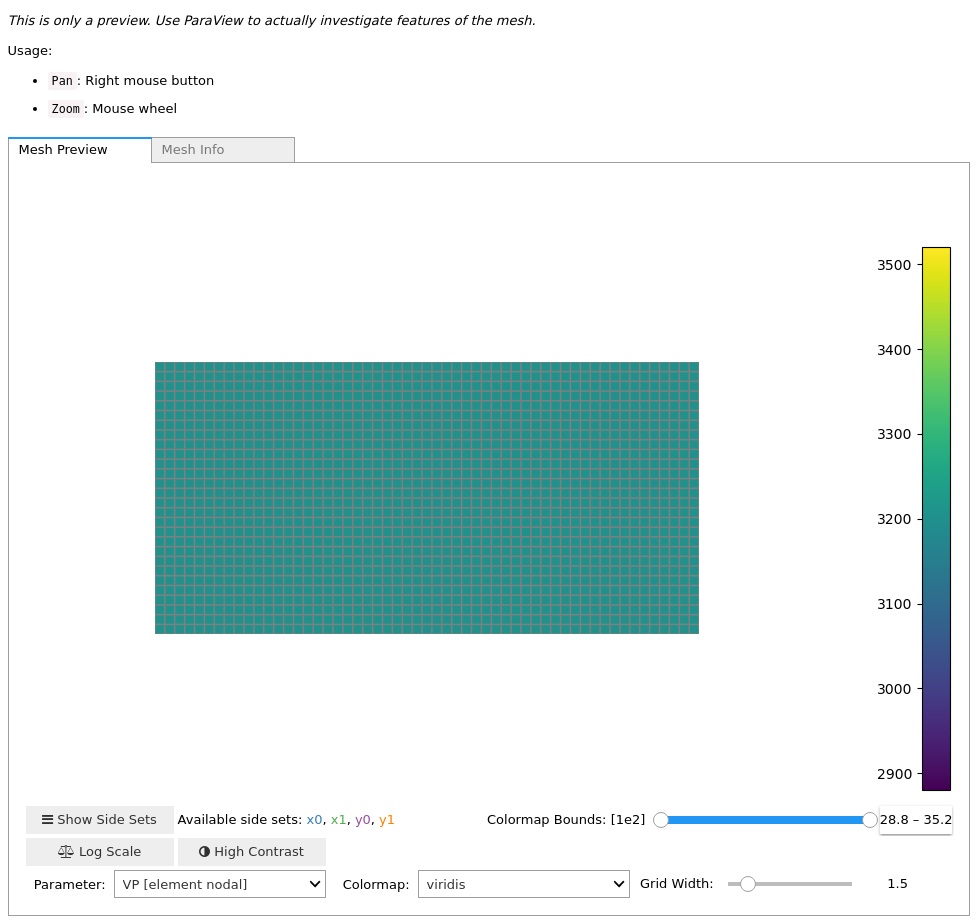<salvus.mesh.unstructured_mesh.UnstructuredMesh at 0x7f828a5b4810>
# Write the mesh to a file which can be visualized in Paraview.
# Open the associated lambs_problem_mesh.xdmf to visualize.
mesh.write_h5("lambs_problem_mesh.h5")

## Setting up a simulation

With our mesh generated we can now go ahead and set up our first simulation.

### Sources and receivers

As a first step we need to set up a source and a few receivers. To faciliate this we'll use several of the objects imported from Salvus Flow above, specifically CartesianVectorSource2D and CartesianReceiver2D. The analytic benchmark we will be comparing to used 2-D vector source with components $[0, -1e10]$ and a relative position of $[1000, 500]$. Here, forces are specified in Newton-meters, with positions in meters. We will choose a Ricker wavelet with a center frequency of $14.5$ Hz as a source time function. For receivers, we'll set up a line of 5 equidistantly spaced between $x=800$ m and $x=1200$ m, at a y position of 1010 m. We need to specify a unique station code for each receiver, as well as a list of which fields we'd like the receivers to record (displacement in this case).

# Sources.
fx, fy = 0.0, -1e10  # Source components (Nm)
sx, sy = 1000.0, 500.0  # Source position (m)
stf = sc.source.stf.Ricker(center_frequency=14.5)  # Center frequency in Hz.
source = sc.source.cartesian.VectorPoint2D(
x=sx, y=sy, fx=fx, fy=fy, source_time_function=stf
)

# Receivers.
nr = 5  # Number of receivers.
ry0 = 800.0  # Receiver y-value.
rx0 = 1010.0  # x-value of first receiver.
rx1 = 1410.0  # x-value of last receiver.
receivers = [
sc.receiver.cartesian.Point2D(
x=x, y=ry0, station_code=f"{_i:03d}", fields=["displacement"]
)
for _i, x in enumerate(np.linspace(rx0, rx1, nr))
]

### Input file generation

We can generate the input options for Salvus Compute. To do this, we'll use the WaveformSimulation object which we imported above. This object helps to encapsulate the myriad of options which we can pass to the solver. We can get started by initializing a new WaveformSimulation object with the mesh, source, and receivers we've generated above. Since we'll be comparing to a semi-analytic solution which was created on a specific time-axis, we also need to explicitly set the start, end, and time step of the simulation to match what is expected, although note that in general the time-step can be automatically determined from the mesh. Finally, when our simulation object is ready to go, we can call the .validate() method which will do some quick checks to ensure that the input file will be accepted by Salvus Compute.

# Set up a waveform simulation object.
sim = sc.simulation.Waveform(mesh=mesh, sources=source, receivers=receivers)

# Modify the start-time, end-time, and time-step of the simulation.
sim.physics.wave_equation.end_time_in_seconds = 0.52
sim.physics.wave_equation.time_step_in_seconds = 1e-3
sim.physics.wave_equation.start_time_in_seconds = -0.08

# sim.output.volume_data.filename = "wavefield.h5"
# sim.output.volume_data.format = "hdf5"
# sim.output.volume_data.fields = ["displacement"]
# sim.output.volume_data.sampling_interval_in_time_steps = 10

# Make sure that Salvus _Compute_ will accept the current options.
sim.validate()

## Running a simulation

Before continuing, ensure that you've completed the necessary Salvus Flow setup as described in the installation instructions at www.salvus.io/documentation/installation/.

With everything ready to go, it's now time to run our first simulation! Salvus Flow's api.run command takes a few arguments worth describing:

• site_name: This is an identifier which tells Flow whether you're running on your local machine, some remote cluster, or perhaps the old chess computer in your grandfather's basement. As long as Salvus has been set up correctly on the specified site all data transfers to / from the local or remote machine will happen automatically. Additionally, if a job management system is present on the remote site Flow will monitor the job queue. Here we're using the site name "local". If you gave your site a different name, please update the site_name parameter below.
• input_file: This expects the WaveformSimluation object which we generated above.
• ranks: This is the number of MPI ranks the job will run on, and can range from 1 to whatever your license will allow.
• output_folder: It is here where all the output files of the simulation will be copied.
• get_all: Salvus can produce a lot of output! The getall flag tells _Flow that we want to fetch everthing which the simlulation creates.

All that's left now is to spin up the simulation on a site of your choosing.

api.run(
input_file=sim,
site_name=SALVUS_FLOW_SITE_NAME,
ranks=4,
wall_time_in_seconds=60,
output_folder="output",
get_all=True,
)
Job job_2102231944593078_f3dcac9193 running on local with 4 rank(s).
Site information:
* Salvus version: 0.11.26
* Floating point size: 32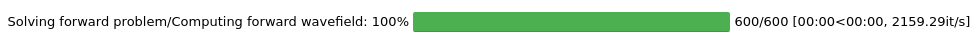* Downloaded 51.6 KB of results to output.
* Total run time: 2.04 seconds.
* Pure simulation time: 0.79 seconds.

<salvus.flow.sites.salvus_job.SalvusJob at 0x7f828e8adf90>

## Matching Lamb's problem

With our first simluation successfully completed, it's now time to take a look at the results. By default Salvus outputs seismograms in the ASDF file format, which can easily be read by Obspy. Below, I've written a simple utility function which takes a Salvus output file, and plots the results against the pre-computed semi-analytical solution.

def plot_asdf_seismograms(asdf_file, axes):
"""
A simple utility function to plot traces side-by side with a
semi-analytic solution to Lamb's problem.
:param asdf_file: ASDF datafile containing receivers.
:param axes: Axes on which to plot.
"""
for i, waveform in enumerate(asdf_file.waveforms):
for j, cmp in enumerate(["X", "Y"]):
trace = waveform.displacement.select(component=cmp)
axes[j, i].plot(trace.data)

for a in axes[:, 0]:
a.set_ylabel("Displacement (m)")
for a in axes[1, :]:
a.set_xlabel("Time sample")

With this function defined, we can now see how well our simulation has done!

fig, axes = plt.subplots(2, 5, sharex="col", sharey="row", figsize=(20, 5))
with ASDFDataSet("force_verticale.h5", mode="r") as ex2ddir, ASDFDataSet(
"output/receivers.h5", mode="r"
) as simdata:
plot_asdf_seismograms(simdata, axes)
plot_asdf_seismograms(ex2ddir, axes)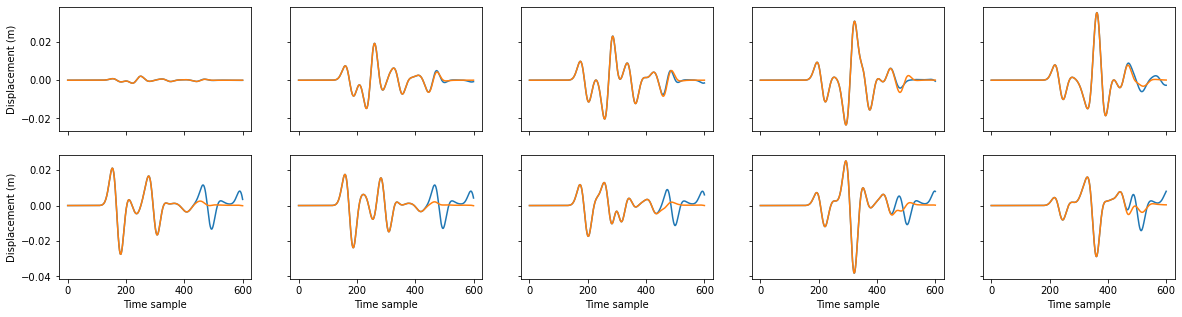Hmm. While we're close, there's something not quite right here. Remember when I mentioned above that we would make the domain infinite later on? Well, now is the time -- the discrepencies at later time are due to reflections off the free-surfaces of the domain. We'll now make our domain "infinite" by adding some absorbing boundaries at the edge of the domain. In this example we will add a simple Clayton-Enquist style boundary condition. While these boundaries do not perform well for waves hitting the boundaries at small angles, they are advantageous in that they do not need any sort of "sponge-layers". We will see how to attach more advanced absorbing boundaries in a future tutorial. For now, we simply need to initialize the AbsobringBoundary object we imported above. To do this, we need to specify at which "side-sets" the boundaries will be applied. By default, Cartesian meshes have side-sets which correspond to the edges of the domain (i.e. $[x0, x1, y0, y1]$ in 2-D, and $[x0, x1, y0, y1, z0, z1]$ in 3-D). Since in this case we want a free surface condition on the top boundary ($y1$), we'll apply the absorbing condition to the other three side sets).

# Initialize first-order absorbing boundary conditions.
boundaries = sc.boundary.Absorbing(
side_sets=["x0", "x1", "y0"], taper_amplitude=0.0, width_in_meters=0.0
)

# Associate boundaries with our simulation.
sim.add_boundary_conditions(boundaries)
sim.validate()

With our boundaries attached, lets now run the simulation again and see if we can match the provided solution this time.

# Run a job.
api.run(
input_file=sim,
site_name=SALVUS_FLOW_SITE_NAME,
ranks=2,
wall_time_in_seconds=60,
output_folder="output",
get_all=True,
overwrite=True,
)

# Plot results.
fig, axes = plt.subplots(2, 5, sharex="col", sharey="row", figsize=(20, 5))
with ASDFDataSet("output/receivers.h5", mode="r") as simdata, ASDFDataSet(
"force_verticale.h5", mode="r"
) as analytic:

# Plot.
plot_asdf_seismograms(analytic, axes)
plot_asdf_seismograms(simdata, axes)

# Ensure seismograms are similar.
for ana, sal in zip(analytic.waveforms, simdata.waveforms):
for cmp in ["X", "Y"]:
ana_trace = ana.displacement.select(component=cmp).data[250:450]
sal_trace = sal.displacement.select(component=cmp).data[250:450]
assert np.linalg.norm(ana_trace - sal_trace) < 1e-3
Job job_2102231944338469_c87408be08 running on local with 2 rank(s).
Site information:
* Salvus version: 0.11.26
* Floating point size: 32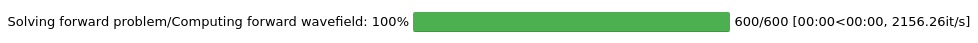* Downloaded 51.9 KB of results to output.
* Total run time: 1.07 seconds.
* Pure simulation time: 0.84 seconds.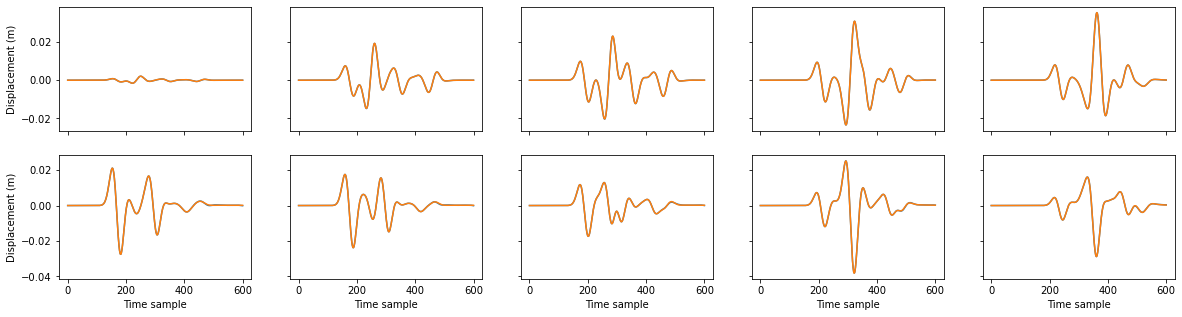Looks like that did it :).

While this is a neat way to introduce some of the simple forward modelling commands, this tutorial has a deeper significance to the Salvus project as a whole. In fact, this very notebook, along with many like it, get run every time a new commit is made to the Salvus codebase. If the solutions do not fit as well as is visualized above, the commit is rejected. This helps us ensure that, as more and more features are added, our solutions remain accurate and performant.

## Under the hood

While SalvusFlow is a great tool for running simulations both large and small, sometimes it doesn't make sense to deal with the overhead of firing up a Python notebook. Fortunatelly, it's quite easy to run SalvusCompute from the command line as well -- all we need to do is generate a toml input file. Let's generate a file from the inputs we've been using so far.

# Write the input file as a toml.
with open("sample_toml_file.toml", "w") as fh:
toml.dump(sim.get_dictionary(), fh)

# Look at the file we've written.
!cat "sample_toml_file.toml"
[domain]
dimension = 2
polynomial_order = 4

[domain.mesh]
filename = "__SALVUS_FLOW_SPECIAL_TEMP__"
format = "hdf5"

[domain.model]
filename = "__SALVUS_FLOW_SPECIAL_TEMP__"
format = "hdf5"

[domain.geometry]
filename = "__SALVUS_FLOW_SPECIAL_TEMP__"
format = "hdf5"

[output.meta_data]
meta_json_filename = "meta.json"
progress_json_filename = "progress.json"

[output.point_data]
filename = "receivers.h5"
format = "asdf"
sampling_interval_in_time_steps = 1
[[output.point_data.receiver]]
location = [ 1010.0, 800.0,]
network_code = "XX"
station_code = "000"
location_code = ""
fields = [ "displacement",]

[[output.point_data.receiver]]
location = [ 1110.0, 800.0,]
network_code = "XX"
station_code = "001"
location_code = ""
fields = [ "displacement",]

[[output.point_data.receiver]]
location = [ 1210.0, 800.0,]
network_code = "XX"
station_code = "002"
location_code = ""
fields = [ "displacement",]

[[output.point_data.receiver]]
location = [ 1310.0, 800.0,]
network_code = "XX"
station_code = "003"
location_code = ""
fields = [ "displacement",]

[[output.point_data.receiver]]
location = [ 1410.0, 800.0,]
network_code = "XX"
station_code = "004"
location_code = ""
fields = [ "displacement",]

[physics.wave_equation]
time_stepping_scheme = "newmark"
start_time_in_seconds = -0.08
end_time_in_seconds = 0.52
time_step_in_seconds = 0.001
courant_number = 0.6
attenuation = false
[[physics.wave_equation.point_source]]
location = [ 1000.0, 500.0,]
spatial_type = "vector"
spatial_weights = [ 0.0, -10000000000.0,]

[physics.wave_equation.point_source.source_time_function]
center_frequency = 14.5
wavelet = "ricker"
[[physics.wave_equation.boundaries]]
side_sets = [ "x0", "x1", "y0",]
taper_amplitude = 0.0
type = "absorbing"
width_in_meters = 0.0



With this input file you can now just run SalvusCompute from the command line, with or without MPI. You can also of course modify the input files, as long as they are consistent with the schema. As with SalvusFlow, most invalid inputs will trigger an informative schema error before the time loop begins.

That's it for this tutorial!PAGE CONTENTS